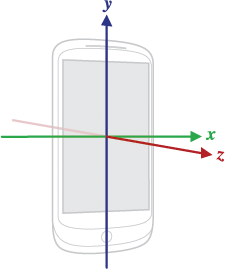## Sunday, 22 February 2015

### Magneto = Gyroscope + Accelerometer + Geomagnetometer

I recently participated in a 24hr hackathon where I built a system called Magneto using which people with physical disabilities will be able to control a computer with arm movements and voice commands. This sort of gives the power of Magneto (from Xmen), hence the name!

Magneto runs on both android phone and wear. Here is a quick view of its working:

If you are interested to view the source. Github: Magneto

The intention of this article is not to showcase Magneto, but to explain the physics behind how I was able to map the sensors on android device to mouse movement. While making it, I had used a lot of builtin functions and some help from FusedGyroscopeSensor. Later, I did a lot of research (mainly on linear algebra and rotation physics) to get a clear understanding of the logic. In this article I will clearly explain the learnings.

#### Sensors

I have used 3 sensors available on android device
1. Accelerometer -  measures the acceleration applied to the device, including the force of gravity.
2. Geo magnetic sensor - lets you monitor changes in the earth's magnetic field.
3. Gyroscope - measures the rate or rotation in rad/s around a device's x, y, and z axis.

#### Getting the initial rotation matrix

The initial rotation matrix describes the initial orientation of the device. It is found using accelerometer (for gravity) and geomagnetic sensor. Any vector when multiplied with this rotation matrix should transform it to world coordinate system. This is an identity matrix if the device is aligned with world's coordinate system, that is, when the device's X axis points toward East, the Y axis points to the North Pole and the device is facing the sky.Device's coordinate system
Step 1 Rotate geomagnetic vector (E) over gravity(g) using Rodrigues' rotation formula

Now normalize the vector

Step 2 Now normalize gravity vector

Step 3 Rotate A over H using Rodrigues' rotation formula

Step 4 The rotation matrix R is represented in terms of H, M and A

#### Get current orientations using gyroscope

Now that we have the initial rotation matrix we can proceed processing the rotations of the device. The gyroscope measures the rate or rotation (not normalized) around a device's x, y, and z axis i.e angular velocity. Also, we keep track of time taken for the device to rotate from its previous position to current position.

Given that gyroscope works very fast, we can divide one action into several smaller samples in a sequence. Now let us consider one such smaller sample.
Say the rates(angular velocity) and time taken are:

"In three-dimensional space, any displacement of a rigid body such that a point on the rigid body remains fixed, is equivalent to a single rotation about some axis that runs through the fixed point."

It means that any rotation can be expressed as a single rotation about some axis. The axis is the unit vector which remains unchanged by the rotation. Let us determine this angle.

Angular speed is calculated. It is just the magnitude of angular velocity.

Angular velocity is normalized. The resultant vector gives us the unit vector in the direction of angular velocity. This is called Euler axis.

Now that we have magnitude  of angular velocity we can calculate Euler angle.

Rotation Vector is a 4 dimensional vector called quaternion represented in terms of Euler axis and angle as follows:

The delta rotation vector is converted to delta rotation matrix. Now, In order to get the resultant rotation matrix, the current rotation matrix is multiplied with the delta rotation matrix.

Now actual angles i.e angles made by the device with respect to device's coordinates (NOT world coordinates) are determined. See Conversion formulae between formalisms.

#### Mapping angles with mouse's coordinates

Now that we have the required angles, we can determine the desired mouse coordinates. If D is the approximate distance of the device from monitor, D tan θ will give its component on the monitor. Using this concept we can find out the desired X and Y coordinates  of the mouse.

Repeat this process (except finding initial rotation matrix of course) every time the device moves to determine mouse coordinates.

NOTE: All formulae were made using Latex and are shared here for your use :)

Sources:
 Android SDK - SensorManager.java